# Create a multivariate basis of functions from scalar multivariable functions¶

## Description¶

We want to build a finite basis of functions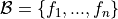where each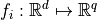with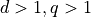, from given scalar multivariable functions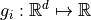.

In other words, we want to create a basis of functions having both a multivariate input and output from functions having a multivariate input and a scalar output.

In this example, we do that by the aggregation of the given functions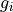, using the AggregatedFunction class. This class allows one to create a multivariate output function from a list of functions having a univariate output dimension. Note that we can only aggregate functions that share the same input space.

We illustrate two cases that differ from the way the functionshave been created:

• Case 1: we suppose that the functionshave no particular origin,

• Case 2: we suppose that the functionshave been built from particular bases.

In both cases, we use the Basis class to create the basis and the AggregatedFunction class to create a multivariate output function from a list of scalar multivariable functions.

## Case 1: Aggregation of given functions¶

In that case, we have some functionsand we aggregate them in order to build the basis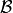.

For example, we set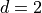,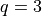and we use the functions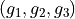defined by: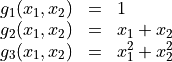and we want to build the finite basis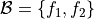defined by: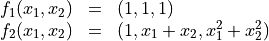import openturns as ot
d = 2
q = 3

# Create the functions g_i
g_1 = ot.SymbolicFunction(['x1', 'x2'], ['1'])
g_2 = ot.SymbolicFunction(['x1', 'x2'], ['x1+x2'])
g_3 = ot.SymbolicFunction(['x1', 'x2'], ['x1^2+x2^2'])

# Create the multivariate basis
f_1 = ot.AggregatedFunction([g_1] * q)
f_2 = ot.AggregatedFunction([g_1, g_2, g_3])
basis = ot.Basis([f_1, f_2])
print('Fonction f_1 : ', basis.build(0))

Fonction f_1 :  [[x1,x2]->,[x1,x2]->,[x1,x2]->]


## Case 2: Aggregation of functions built from given bases¶

In that case, we use some functionsthat have been built from particular bases. For example, they are members of polynomial families which are orthonormal to given distributions.

In this example, we use both polynomial families:

•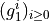which is a orthonormal multivariate polynomial basis with respect to the uniform distributipn on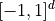(it is obtained through a tensor product of univariate Legendre polynomials orthonormal with respect to the uniform distribution on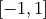),

•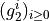which is a orthonormal multivariate polynomial basis with respect to the multivariate standard normal distribution (it is obtained through a tensor product of univariate Hermite polynomials orthonormal with respect to the standard normal univariate distribution).

We consider the linear enumeration of the polynomial family. The functions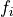are built as the aggregation of the-th polynomials of the families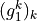and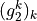. We only consider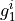and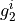of total degree less than 2: then we have 6 polynomials.

We still set,.

d = 2

legendre_builder = ot.OrthogonalProductPolynomialFactory([ot.LegendreFactory()] * d)
hermite_builder = ot.OrthogonalProductPolynomialFactory([ot.HermiteFactory()] * d)

# Generate the multivariate functions f_i (quadratic)
total_deg = 2
basis_size = ot.LinearEnumerateFunction(d).getStrataCumulatedCardinal(total_deg)
basis = []
for i in range(basis_size):
f_i = ot.AggregatedFunction([legendre_builder.build(i), hermite_builder.build(i)])
basis.append(f_i)

basis = ot.Basis(basis)